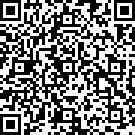### 问题分类 [C]

0

1

431

``````#include<stdio.h>
#include<time.h>
#include<stdlib.h>
#include <graphics.h>
int r={{30,20,130,60},{170,20,220,60},{260,20,310,60}};//三个按钮的二维数组
int guess,num;
char s;//输入字符串变量
int button_judge(int x,int y)//按钮判断函数
{
if(x>r &am``````

0

1

817

-1

1

522

``````void setmap()
{
char num;
int days;
int n[NUM];
int q=0;
double a[NUM];
InputBox(num, 50, _T("请输入测试数:<50"));
int b;
settextcolor(LIGHTBLUE);
b = atoi(num);
int k = 0;
while (b--)
{
char e, r, t;
struct map* df;
df = (map*)malloc(si``````

0

2

584

``````#include<stdio.h>
#inclu``````

0

1

387

HWND hwnd = CreateWindow(a.lpszClassName,a.lpszClassName, WS_OVERLAPPEDWINDOW, CW_USEDEFAULT, CW_USEDEFAULT, CW_USEDEFAULT, CW_USEDEFAULT, NULL, NULL, hinstance, NULL);

0

1

474

outtextxy输出链表数据域中的字符串乱码

typedef struct student{char data;}student;

typedef struct Node{student Sdata;Node* next;}Node;

0

1

755

easyX为何我这个代码实现不了多个图片构成一个动画的效果

``````#include <stdio.h>
#include<graphics.h>
#include<conio.h>
int main()
{
for (i = 0; i < 4; i++)
{
swprintf_s(file, L"./debug/zhujued%d.png", i+1);

1

1

1399

``````#include <stdio.h>

int main()
{
IMAGE zhujuea;
IMAGE zhujues;
IMAGE zhujued;
IMAGE zhujuew;
``````

0

1

720

liveRPG.h

``````#pragma once

#include <stdi``````

0

1

728

``````void  Color_test(IMAGE* pimg)
{
DWORD* p = GetImageBuffer(pimg);	// 获取显示缓冲区指针
COLORREF c;
float* h;
float* s;
float* v;
for (int i = pimg-&``````

0

1

1320

putimage()显示图片，图片移动后原来留下来的图怎么处理，才能消失
``````
#include <graphics.h>
#include <time.h>
#include <conio.h>
#include <ctype.h>
#include <iostream>

using namespace std;

#define MAXSTAR 600	// 星星总数

struct STAR
{
double	x;
int		y;
double	step;
int		color;
};

str``````

0

1

2288

0

1

771

``````void printall（）
{
putimage(0, 0, &playlist);///
int position_x = 476;//“序号”横坐标
int position_y = 92;//“序号&r``````

0

1

738

0

1

950

``````void times()
{

int times=360;
TCHAR s;
while(times>=0)
{
system("cls");
LOGFONT f;
gettextstyle(&f);
f.lfHeight =20;
_tcscpy(f.lfFaceName, _T("黑体"));
f.lfQual``````

0

1

788

C语言新手问题

0

1

1267

``node->val = root->val;``

``````/***

0

1

748

``````/****

***/
#include<stdio.h>
#include<malloc.h>
typedef struct TreeNode {
struct TreeNode* left;
struct TreeNode* right;
int val;
}BiTree;
/**

``````

0

1

784

``````string robot_name= "你好";
initgraph(1000, 800);
setbkcolor(WHITE);
setlinecolor(BLUE);
settextcolor(BLUE);
cleardevice();
rectangle(10, 10, getmaxx() - 10, getmaxy() - 10);
RECT girlname={ 50,50,450,100 };
string girl_name = robot_name;
drawtext((LPCTSTR)girl_``````

0

1

1306

``````#include<stdio.h>
#include<math.h>
int main()
{
int i = 1, sign``````

1059085 (C语言革命0)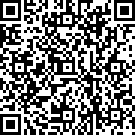8086730 (C语言革命1)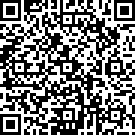2861971 (C语言革命2)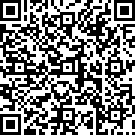19430220 (C语言革命6)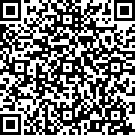46612969 (C语言革命7)